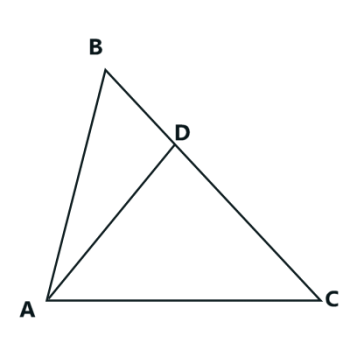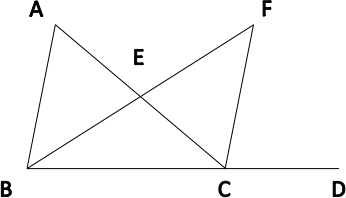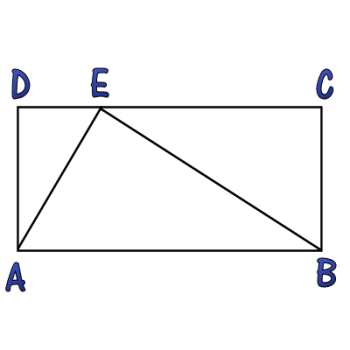Games
Problems
Go Pro!

# Split Triangle

Pro Problems > Math > Geometry > Triangles

## Split Triangle

Segment AD is drawn in triangle ABC, with D on side BC. Given the following information, determine the measure of angle ADB.measure of angle BAD = x
measure of angle CAD = 2x
measure of angle ADC = 2y
measure of angle ABD = y
measure of angle ACD = x + y

Presentation mode
Problem by BogusBoy

## Solution

In order to make it feasible for teachers to use these problems in their classwork, no solutions are publicly visible, so students cannot simply look up the answers. If you would like to view the solutions to these problems, you must have a Virtual Classroom subscription.Assign this problem

## Similar Problems

### Triangle Variables

An isosceles triangle is a triangle in which two of the angles have the same measure. In an isosceles triangle, the angle measures are 30 + x, 62 - x, and 10 + 2x + y. What are the possible numeric values for the measures of the angles?

### Three Two-Digit Angle Measures

The measures of the angles in a triangle are all two digit numbers, and two of them end in 3. Exactly two of them contain the digit 4. None of the angle measures are multiples of three. What are the measures of the angles?

The angles in a triangle have measures x2 - 5, 2x + 18, and x + 37. What is the measure of the largest angle in the triangle?

### Three Triangles

The angles in a triangle have measures x, x + 10, and y.

The angles in a second triangle have measures x + y, 40, and 50.

The angles in a third triangle have measures x – y, 40, and z.

What is the value of z?

### Arithmetic Angles

The angles of a triangle are in arithmetic progression, and the difference between the largest and smallest angle is 42 degrees. What are the three angles?

### Triangle in a Square

Triangle ABC is inside square ABDE, and C lies along side DE. If the area of the triangle is 24 square units, what is the length of AB?

### Angle Bisector

In the diagram shown,m ∠DCF = 80
m ∠ACF = 30
m ∠FBD = 50.

If segment BF bisects  ∠ABD, find m ∠BAC.

### Triangle and RectangleIn the diagram displayed, the triangle splits the rectangle into three similar triangles. Find the value of AD2 divided by the product of DE and EC.

### Angle Measure

Line segment AB is intersected by segment DC, with D on AB, between A and B.

In triangle ADC, m∠ADC = 30º + x and  m∠DAC = 50º

In triangle ABC, m∠ACB = 115º + x

In triangle CDB, m∠CDB = 140º + x

Find m∠CBD.

### Triangle in a Square

A triangle is placed on the interior of a square so that two of the triangle's vertices lie on two adjacent vertices of the square, and the third vertex lies on the opposite side,
1
3
of the way between the two endpoints.

If the perimeter of the triangle (in linear units) is equal to the area of the triangle (in square units), what is the length of the side of the square?

Forty-five and Thirty, Two Triangles with Angle Measures, Angles in a Triangle, Angle Riddle# Blogs on This SiteReviews and book lists - books we love!The site administrator fields questions from visitors.Like us on Facebook to get updates about new resources